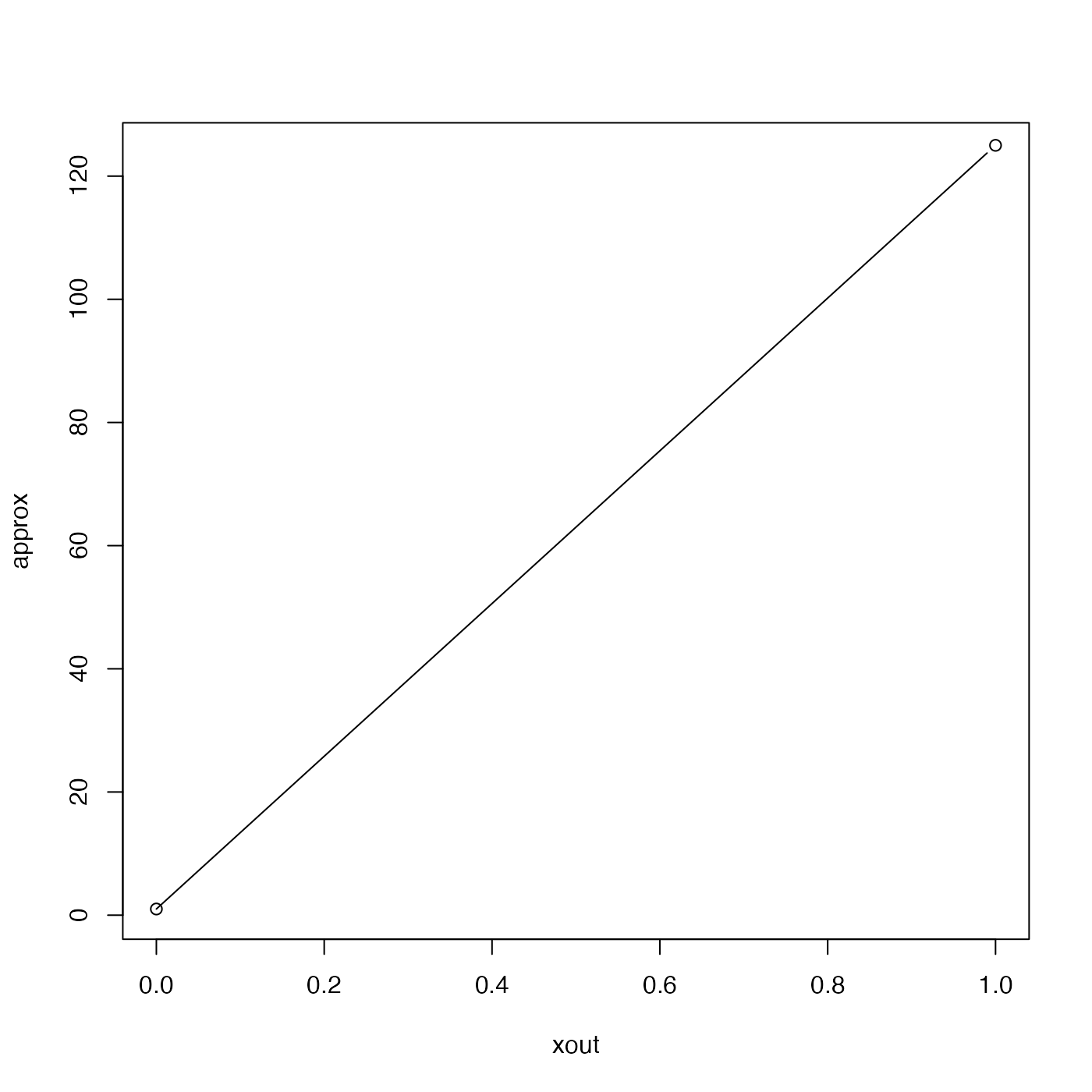Interpolate within a 3D array, using the trilinear approximation.

approx3d(x, y, z, f, xout, yout, zout)

## Arguments

x vector of x values for grid (must be equi-spaced) vector of y values for grid (must be equi-spaced) vector of z values for grid (must be equi-spaced) matrix of rank 3, with the gridded values mapping to the x values (first index of f), etc. vector of x values for output. vector of y values for output (length must match that of xout). vector of z values for output (length must match that of xout).

## Value

A vector of interpolated values (or NA values), with length matching that of xout.

## Details

Trilinear interpolation is used to interpolate within the f array, for those (xout, yout and zout) triplets that are inside the region specified by x, y and z. Triplets that lie outside the range of x, y or z result in NA values.

## Examples

## set up a grid
library(oce)
n <- 5
x <- seq(0, 1, length.out=n)
y <- seq(0, 1, length.out=n)
z <- seq(0, 1, length.out=n)
f <- array(1:n^3, dim=c(length(x), length(y), length(z)))
## interpolate along a diagonal line
m <- 100
xout <- seq(0, 1, length.out=m)
yout <- seq(0, 1, length.out=m)
zout <- seq(0, 1, length.out=m)
approx <- approx3d(x, y, z, f, xout, yout, zout)
## graph the results
plot(xout, approx, type='l')points(xout, f[1, 1, 1])points(xout[m], f[n,n,n])